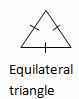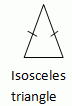Email us to get an instant 20% discount on highly effective K-12 Math & English kwizNET Programs!

#### Online Quiz (WorksheetABCD)

Questions Per Quiz = 2 4 6 8 10

### MEAP Preparation - Grade 7 Mathematics4.16 Classifying Triangles by Sides

#### Classification based on sides

Triangles can be divided into three kinds according to the measures of their sides.
1. Equilateral triangle:
• A triangle whose sides are equal is called an equilateral triangle.
• All the angles of an equilateral triangle are equal.2. Isosceles triangle:
• A triangle in which two sides are equal is called an isosceles triangle.
• In an isosceles triangle the unequal side is called the base of the triangle.
• The base angles of an isosceles triangle are congruent.3. Scalene triangle:
• If no two sides of a triangle are equal, it is called a scalene triangle.Directions: Read the above properties of triangles and answer the following questions:
1. Illustrate each of the above properties by drawing a triangle.
2. Write in your own words, the different classification of triangles based on sides.

 Q 1: In a triangle, the sides are given as 6cm, 6cm and 4cm. Which of the following best describes this triangle.Scalene triangleEquilateral TriangleIsosceles Triangle Q 2: If the sides of a triangle are given by 6 cm, 5 cm and 7 cm, then the triangle is a _________ triangle.Answer: Q 3: "In an equilateral triangle all the sides are _________". Answer: Q 4: "The base angles of an isosceles triangle are congruent".FalseTrue Q 5: If the angles of a triangle in degree measure are 60, 120/2 and 180/3, then it is a (an) _________ triangle.Answer: Q 6: Name the triangle whose sides are given by 10'', 10'' and 10''.Scalene TriangleEquilateral TriangleIsosceles Triangle Q 7: If the sides of a triangle are of lengths 45/3 cm, 24/2 cm and 30/2 cm, then the triangle is a (an) _________ triangle.Answer: Q 8: In a (an) _________ triangle the unequal side is called the base of the triangle equilateralscaleneisosceles Question 9: This question is available to subscribers only! Question 10: This question is available to subscribers only!